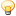A Flash Developer Resource Site

# Thread: 21 photos combinations, a maths question

1. ## 21 photos combinations, a maths question

I'm a little stuck on the maths of this puzzle. Anybody know?

I have 21 photos and I've given them to a few dozen different people. Each person can order them how they like and use as many or as little as they wish, but may not use the same one twice.

How many different combinations are there?Reply With Quote

2. y number of people
x number of combinations per person
u number of combos total
x * y = u

some info that is needed is what do you mean may not use the same one twice.

if you send one person 21 pictures, he has at the most 21 different combinations if he can only use a single picture one time.

so that would make it

21*Y=U

but if you are talking combinations of photos such as, they cant use pictures 1 and 3 together more then once, then it turns into

21+20+19+18+17+16+15+14+13+12+11+10+9+8+7+6+5+4+3+ 2+1=x (i dont know how to make the correct mathmatical symbol in a forum)Reply With Quote

3. ##You have to use combinations. The first number is the amount of things to choose from, the second is amount of things you can choose:

21 combo 1 (the possibilities for choosing 1 photo = 21) +
21 combo 2 (=210) +
21 combo 3 (=1330) +
21 combo 4 (=5985) +
21 combo 5 (=20349) +
21 combo 6 (=54264) +
21 combo 7 (=116280) +
21 combo 8 (=203490) +
21 combo 9 (=293930) +
21 combo 10 (=352716) +
21 combo 11 (=352716) +
21 combo 12 (=293930) +
21 combo 13 (=203490) +
21 combo 14 (=116280) +
21 combo 15 (=54264) +
21 combo 16 (=20349) +
21 combo 17 (=5985)
21 combo 18 (=1330) +
21 combo 19 (=210) +
21 combo 20 (=21) +
21 combo 21 (=1)

Add them all up : 2,097,130
I'm pretty sure on this, as long as I did not make a mistake adding. I used This tool to calculate combinations

-jtnwReply With Quote

4. You can think of it as a summation series of combinations.

nCr is the number of ways of choosing r things from a total of n
so here n=21

if people can choose as many photos they like up to a total of 21, and as few as 1 photo
then we have the series

21C21 + 21C20 + 21C19 ... 21C2 + 21C1 + 21C0

or

nCn + nC(n-1) + nC(n-2) ...

A series like this converges to equal 2^n

Now because we dont want to count the option where no photos are taken the answer is (2^n) - n

or in this case = 2,097,131

Thats my take, its been years since I've done any prob theory so I might be wrongReply With Quote

5. much better clean math and explanation ctranter!Reply With Quote

6. our answers are one different. annoying!i hope its close enough for asheep

actually I think it should be (2^n) - 1

as 21C0 is of course 1 not 21

and hence 2,097,151

hmmmmmReply With QuoteReply With Quote

21
210
1330
5985
20349
54264
116280
203490
293930
352716
352716
293930
203490
116280
54264
20349
5985
1330
210
21
1 +
--------
2097151Reply With Quote

9. Amazing guys. I had 5,842,587,018,598,200,000,000,000,000 or 441. So yeah, nice one!

Thanks.Reply With Quote

10. Crap, now I need to work out how many combinations there are if you're allowed to use the same photo more than once (so I can compare...)

That must be quite simple?Reply With Quote

11. You could use this tool and set order important to no, repetition allowed to yes and brute add the way I did. Or maybe ctranter will remember some convergent formula...Reply With Quote

12. If I do that I end up with 5,842,587,018,598,200,000,000,000,000 (or 5.84258701838598e+27) Formula being n^r. That seems like a big number though...Reply With Quote

13. asheep what do you mean by combinations?Reply With Quote

14. did you make sure to set order important to no--I'm assuming that's what you want.

I'm bored (and this took < 5 min) so I wrote this code:
Code:
```//Factorial function from flash help
_global.factorial = function(n:Number) {
if(n <= 1) {
return 1;
}
else {
return n * factorial(n - 1);
}
}

num=0
n=21
for(var r=1; r<=n; r++) {
num+=factorial(n+r-1)/(factorial(r)*factorial(n-1))
}
trace(num)```
I get 538,257,874,439

-jReply With Quote

15. I make the summation series to be:Which my calculator says evaluates to 538,257,874,439 (5.38257874439x10^11)Reply With Quote

16. once again, seconds apart with slightly different answers :/Reply With Quote

17. My calc didn't give sufficient d.p. so I re-ran it on the pc and got exactly the sameReply With Quote

18.Reply With Quote

19. you guys are way to fing smart to be hanging around here (except silver of course)Reply With Quote

20. I love the use of Flash, brilliant.

Thanks a million guys, appreciated!Reply With Quote

maths geeks####Posting Permissions

• You may not post new threads
• You may not post replies
• You may not post attachments
• You may not edit your posts
•

 » Home » Movies » Tutorials » Submissions » Board » Links » Reviews » Feedback » Gallery » Fonts » The Lounge » Sound Loops » Sound FX » About FK » Sitemap